## Word Problems

Practice Unlimited Questions

#### 1. Ehi has a watermelon, a papaya and a mango. The mass of the watermelon is three times the mass of the papaya. The mass of the mango is half the mass of the papaya. < If the three fruits have a mass of 5850 grams, what is the mass of the watermelon? Express your answer in kilograms and grams.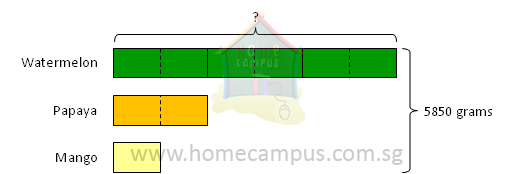9 units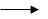5850 grams
1 unit5850  ÷  9  =  650 grams
6 units650  ×  6  =  3900 grams  =  3 kg 900 grams

The mass of the watermelon is 3 kg 900 grams.

#### 2. Amar had 216 more marbles than Ajay. Amar had 3 times as many marbles as Ajay. a. How many marbles had the two boys altogether? b. Amar gave some marbles to Ajay. In the end, the two boys had an equal number of marbles. How many marbles did Amar give to Ajay?

a. Before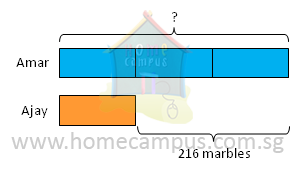2 units216 marbles
1 unit216  ÷  2  =  108 marbles
4 units108  ×  4  =  432 marbles

The two boys had 432 marbles altogether.
b. After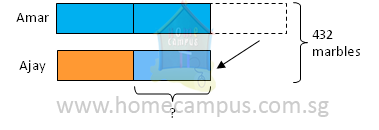1 unit108 marbles
Amar gave 108 marbles to Ajay.

#### 3. 2358 people went to watch a 3D movie. There were 496 more children than adults. If there were 185 more girls than boys, how many girls went to watch the movie?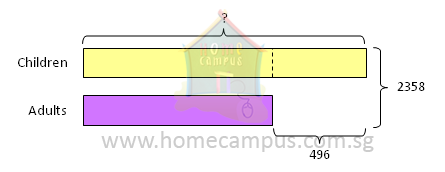2358  −  496  =  1862
2 units1862
1 unit1862  ÷  2  =  931
931  +  496  =  1427

There were 1427 children.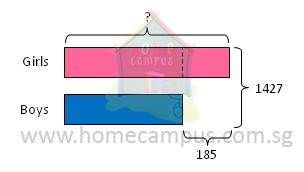1427  −  185  =  1242
2 units1242
1 unit1242  ÷  2  =  621
621  +  185  =  806

806 girls went to watch the movie.

#### 4. 3 cartons have 3072 crayons altogether. Each carton has an equal number of packets. Each packet contains 8 crayons. Find the number of packets in each carton.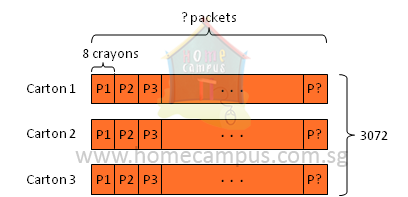3072  ÷  3  =  1024
There are 1024 crayons in each carton.

1024  ÷  8  =  128
There are 128 packets in each carton.

#### 5. A farmer picks 75 kg of apples a day. He keeps 6 kg of apples for himself each day and sells the rest. Find the mass of apples he will sell in July.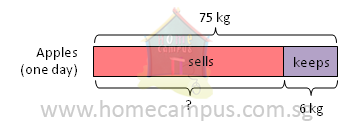75  −  6  =  69
He sells 69 kg of apples a day.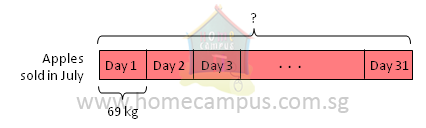69  ×  31  =  2139
He will sell 2139 kg of apples in July.
There are 31 days in July.

#### 6. Anna, Bella and Clara went to a sports shop and bought similar tennis paraphernalia. Anna paid \$182 for 2 tennis rackets and 6 tennis balls. Bella paid \$340 for 4 tennis rackets and 6 tennis balls. If Clara bought 6 tennis rackets, how much did she pay?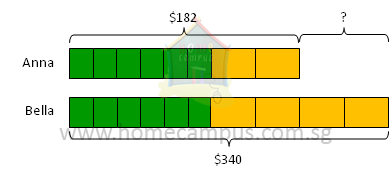\$340  −  \$182  =  \$158
2 units\$158
1 unit\$158  ÷  2  =  \$79

Each tennis racket costs \$79.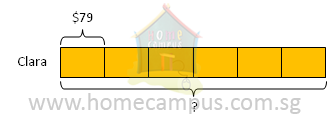\$79  ×  6  =  \$474
Clara paid \$474 for 6 tennis rackets.

#### 7. Jean used 18 m of cloth to make 4 similar curtains and 6 similar cushion covers. She used 3 m of cloth to make each curtain. How much cloth did she use to make each cushion cover?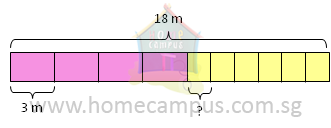3 m  ×  4  =  12
She used 12 m of cloth on all the curtains together.

18 m  −  12 m  =  6 m
She used 6 m of cloth on all the cushion covers together.

6 m  ÷  6  =  1
She used 1 m of cloth to make each cusion cover.

#### 8. Ken and Ben had 132 paper clips altogether. Ken gave away 17 paper clips to Ben. In the end, Ken had 3 times as many paper clips as Ben. a. How many paper clips did Ken have in the end? b. How many paper clips did Ben have in the beginning?

a. After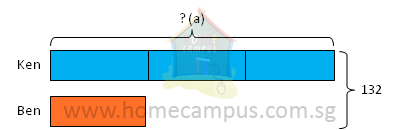4 units132 paper clips
1 unit132  ÷  4  =  33 paper clips
3 units33  ×  3  =  99 paper clips

Ken had 99 paper clips in the end.
b. Before
33  −  17  =  16
Ben had 16 paper clips in the beginning.